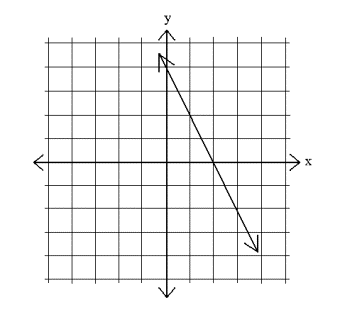# Writing a linear equation

An algebraic equation in which each element (or term) is either a constant or the product of the first power of a single variable and a constant is called a linear equation. These equations can have one or more variables.

Depending on the number of variables (we will mark that number with the letter n), a linear equation is the algebraic representation of a straight line in a coordinate system with n dimensions. If we are talking about the usual two-dimensional Cartesian coordinate system, a linear equation will consist of two variables (usually x and y), the slope and the intercept.  The slope tells how much the value of one variable changes if the other one changes by a single unit. If you are calculating the value of y, the intercept shows at which point the line crosses the y-axis.

## How to write a linear equation

There are several different forms of linear equations that can be converted from one to another using the basic laws of elementary algebra. We will explain the ones that are most often used in practical mathematics.

### The general form

Depending on the number of variables (if we represent the variables with x1, x2, x3…, xn and the constants with a1, a2, a3… an and b), the general form of a linear equation looks like this:

a1*x1 + a2*x2 + …. + an* xn + b = 0

The most commonly used general form of a linear equation (in two-dimensional coordinate systems) is:

a*x + b*y +c = 0### The standard form

This is a very commonly used form of a linear equation as it appears frequently in mathematical textbooks.  It generally looks like this:

a*x + b*y = C

The value of both a and b cannot be equal to zero –at least one of them has to be positive. It can be converted to the general form, but not always to other forms of equations if the value of a or b is equal to zero.

### The slope-intercept form

This is also a very commonly used form of linear equations. It is called the slope-intercept form because both slope and the intercept of a line can be simply read from equations expressed in this form.  It looks like this:

y = mx + b

Here the letter m in front of the variable x represents the slope of the line and b is the value of the intercept on the y-axis. The important thing to remember is that equations of vertical lines cannot be expressed in this form, as their slope is undefined.

These are the basic forms of linear equations. Now, to write down a linear equation in one of these forms, keep a few things in mind. First, it helps if you have a visual representation of the linear equation you are trying to write down. So, if you are given two or more points through which your line passes, draw a graph first. Secondly, try finding the intercepts and the slope. They are generally easy to find and it is easy to convert a slope-intercept equation into most other forms.

It might seem a bit complicated now, but with some practice it will become all too easy. To practice the skill of writing a linear equation, please use the math worksheets below.

## Writing a linear equation exams for teachers

 Exam Name File Size Downloads Upload date Slope Writing a linear equation – Slope – very easy 667.9 kB 7267 September 3, 2019 Writing a linear equation – Slope – easy 894.8 kB 5147 September 3, 2019 Writing a linear equation – Slope – medium 458.2 kB 7368 September 3, 2019 Writing a linear equation – Slope – hard 461.6 kB 5525 September 3, 2019 Fundamental Writing a linear equation – Fundamental equations – very easy 460.4 kB 5944 September 3, 2019 Writing a linear equation – Fundamental equations – easy 12.5 kB 4690 January 20, 2012 Writing a linear equation – Fundamental equations – medium 784.2 kB 5352 September 3, 2019 Writing a linear equation – Fundamental equations – hard 462.4 kB 3823 September 3, 2019 Writing a linear equation – Fundamental equations – very hard 460.1 kB 3346 September 3, 2019 Standard Writing a linear equation – Standard – very easy 459.4 kB 4899 September 3, 2019 Writing a linear equation – Standard – easy 462.6 kB 4013 September 3, 2019 Writing a linear equation – Standard – medium 784.5 kB 4407 September 3, 2019 Writing a linear equation – Standard – hard 460.1 kB 3432 September 3, 2019 Writing a linear equation – Standard – very hard 459.5 kB 3221 September 3, 2019

## Writing a linear equation worksheets for students

 Worksheet Name File Size Downloads Upload date Writing a linear equation – Slope 2 MB 6459 September 3, 2019 Writing a linear equation – Fundamental 550.8 kB 5825 September 3, 2019 Writing a linear equation – Standard 544.7 kB 4847 September 3, 2019